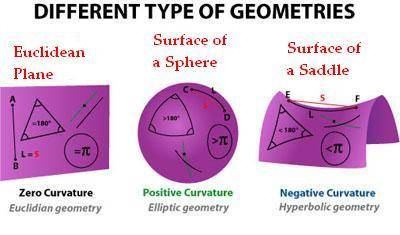# Non-Euclidean geometry help

• A
• btb4198

#### btb4198

In the book: The Elements Euclid defined 5 postulates:

1) A straight line segment can be drawn joining any two points.
2) Any straight line segment can be extended indefinitely in a straight line
3) Given any straight line segment, a circle can be drawn having the segment as radius and one endpoint as center.
4) All right angles are congruent.
5) If two lines are drawn which intersect a third in such a way that the sum of the inner angles on one side is less than two right angles, then the two lines inevitably must intersect each other on that side if extended far enough.
This postulate is equivalent to what is known as the parallel postulate.

can someone please explain to me how :

anything in that video breaks any of the 5 postulates of Euclidean geometry?

What makes it non-Euclidean geometry?

the first example is a tunnel that is really long from the outside but it's actually really short on the inside.

Which of the 5 postulates does this break?
definitely not 1, 2, or 3 unless I can crazy
maybe 4 by I am not sure

and unless I missed something, I thought postulate 5 unprovable.

Last edited by a moderator:
I would say in the room example, it looks like three 90 degree turns are made to return back to the same place whereas in a Euclidean world it would take four 90 degree turns to get back to the same place.

The three 90 degree turns is reminiscent of walking on a sphere:I'm not sure about the remaining examples other than they clearly violate our notions of reality and of course are just renderings controlled by the Unity game engine and how the developer designed the spaces.

As he mentions in the video, his model was the Escher staircase painting which more of an optical illusion than a true non-euclidean world. Escher's painting was based on Penrose steps:https://en.wikipedia.org/wiki/Penrose_stairs

Based on the description the stairs can't exist in a Euclidean world. However, that doesn't mean it exists in a non-Euclidean world but is rather an illusion of the mind.

Last edited:
It's almost always the parallel postulate. That's what guarantees that the interior angle sum is the value of Euclidean geometry, and if you violate that you get curved space.

You can also ask what "straight" means in (1), which is an interesting question on its own and leads to different geometries.

Consider the following situation: There is a "strait line" and point which is not on that line.
How many "strait lines" are passing trough the point and doesn't touch the first strait line (parallel to it).

Every part of the "strait line" between two points is shortest path , depending of the space , between those two points.
There are three possibilities :1. Euclidian geometry
- also known as flat, with zero curvature
- exactly one parallel strait line
- sum of angles in the triangle is 180 degrees
- π is 3.14... ratio

2. Reimann geometry ( German mathematician Bernhard Reimann )
- also known as spherical, elliptic, with positive curvature
- There is no parallel lines / all "strait lines" (big circles) are intersect on opposite sides of the "universe" (sphere)
- sum of angles in the triangle is greater then 180 degrees
- π is 3.14...

3. Lobachevski /Bolai geometry
- also known as hyperbolic, on saddle , with negative curvature
- infinite number of parallel lines (two of them are "hyper parallel" )
...